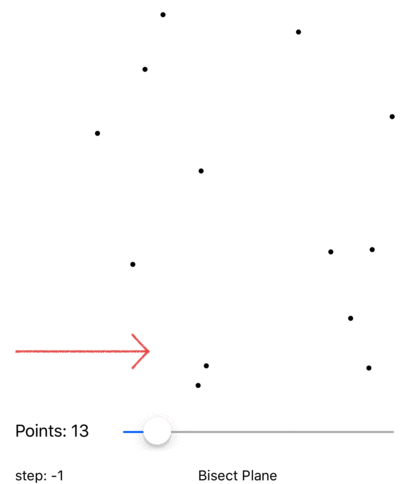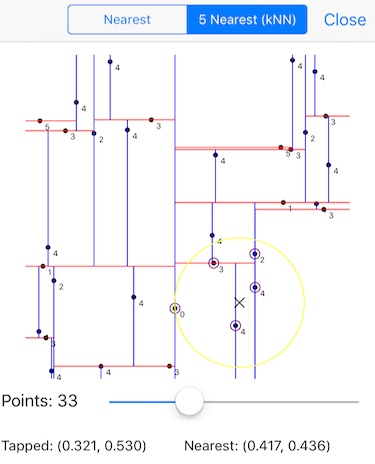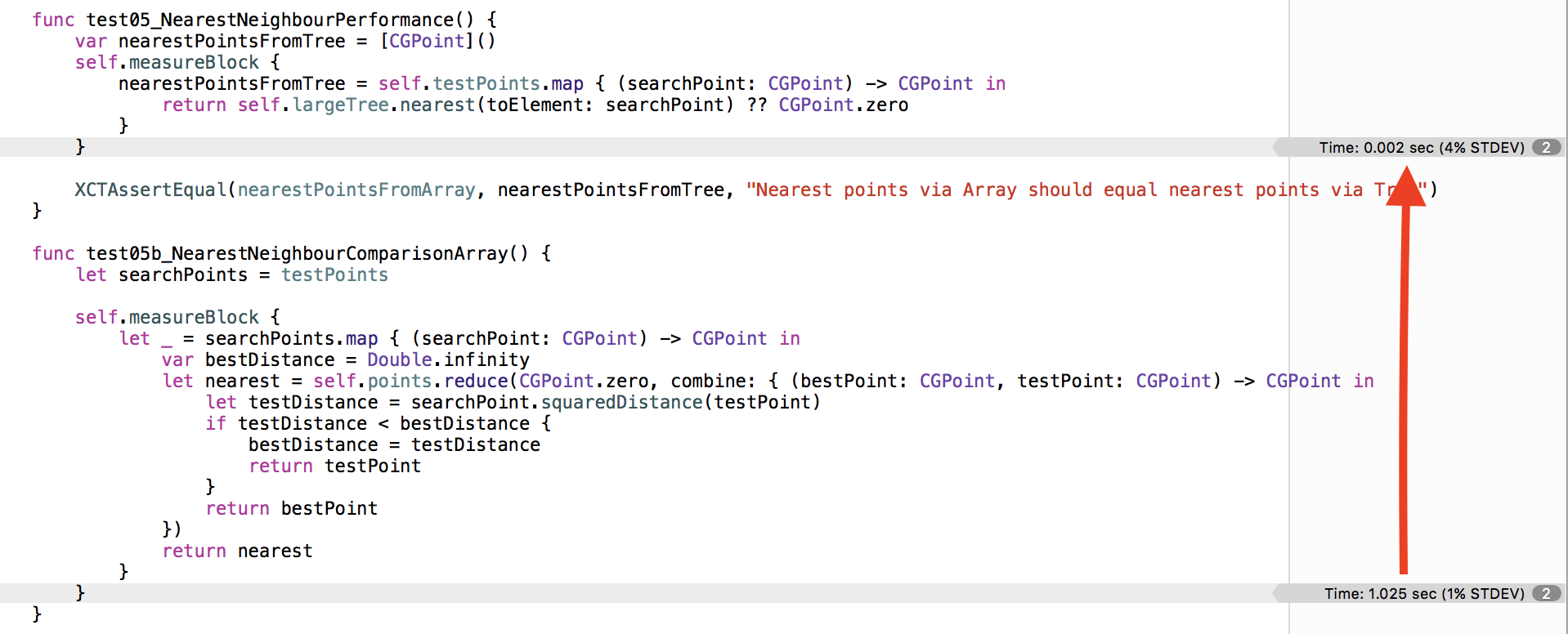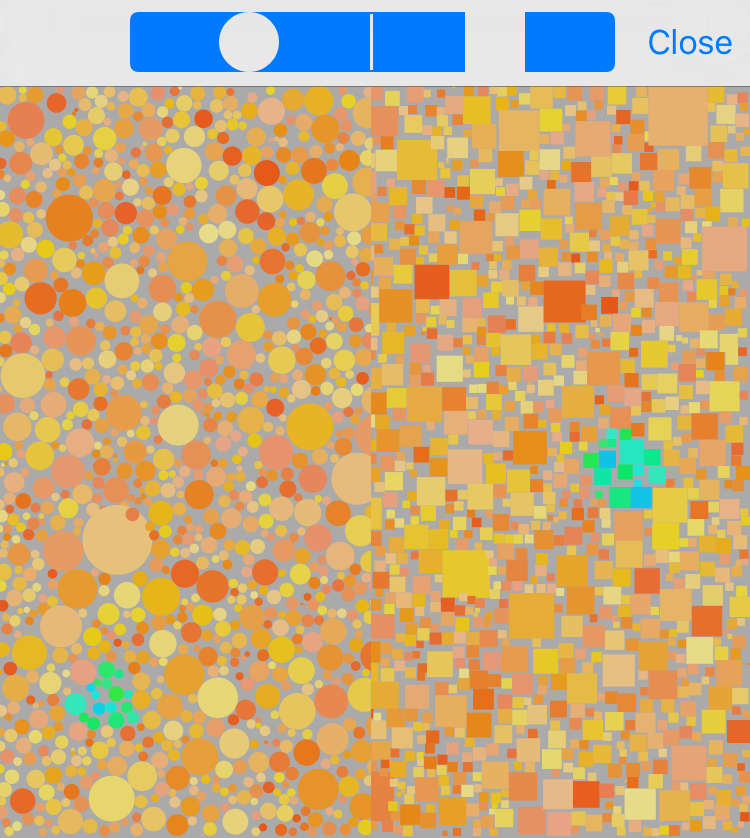Swiftpack.co - Package - Bersaelor/KDTree

# KDTree

Swift implementation of a k-dimensional binary space partitioning tree. The KDTree is implemented as an immutable enum, inspired by functional trees from objc.io. KDTree algorithm according to Wikipedia and ubilabs js example.

If you want to know more, you can watch this talk I gave at funswiftconf.

Visual animation of creating a KDTree:Very simply put, a tree is created by:

1. find the median point in the current direction
2. bisect along the hyperplane(=line in 2D) going through the current median
3. go one step deeper, same thing with next axis

The blue lines go through nodes that partition the plane vertically, the red ones for horizontal partitions.

## Usage

Import the package in your *.swift file:

``````import KDTree
``````

Make sure your data values conforom to

``````public protocol KDTreePoint: Equatable {
static var dimensions: Int { get }
func kdDimension(_ dimension: Int) -> Double
func squaredDistance(to otherPoint: Self) -> Double
}
``````

(CGPoint conforms to KDTreePoint as part of the package)

Then you can grow your own Tree:

``````extension CustomDataPoint: KDTreePoint { ... }

let dataValues: [CustomDataPoint] = ...

var tree: KDTree<CGPoint> = KDTree(values: dataValues)
``````

Then you can `insert()`, `remove()`, `map()`, `filter()`, `reduce()` and `forEach()` on this tree with the expected results, as KDTree conforms to Sequence.

## Applications

### K-Nearest Neighbour:Given a KDTree:

``````var tree: KDTree<CGPoint> = KDTree(values: points)
``````

we can retrieve the nearest Neighbour to a test point like so

``````let nearest: CGPoint? = tree.nearest(to: point)
``````

or the get the 10 nearest neighbours

``````let nearestPoints: [CGPoint] = tree.nearestK(10, to: point)
``````

Complexity is O(log N), while brute-force searching through an Array is of cource O(N).

Preliminary performance results can be gained by running the unit tests, the load example has 10.000 random points in [-1,1]x[-1,1] and find the nearest points for 500 test points:### Tesselations:### Range-Search:

It's also possible to use the KDTree to search for a range of values using:

``````let pointsInRange: [CGPoint] = tree.elementsInRange([(0.2, 0.4), (0.45, 0.75)])
``````

One example is based on the HYG Database of 120 thousand stars. Here we see a piece of the sky around 10h right ascension and 35° declination where the KDTree algorithm can be used to both get the stars in the area the iPhone screen is pointing at and also find the closest Star to a position the user is tapping via NN:

## Examples of Implementation

The example app in the `Example` folder shows some demo implementations on `iOS`.

`KDTree` can also be used as a package in a server-side-swift app. StarsOnKitura is one example that returns stars from the HYG database of 120'000 stars using `ascension`/`declination` parameters. Live available running on a 64MB instance on IBM Bluemix.

## Installation

In Xcode, open `File` -> `Swift Packages` -> `Add Package Dependency` and copy the project url into the text field:

``````https://github.com/Bersaelor/KDTree
``````

Or add the following to your `Package.swift` dependencies

``````.Package(url: "https://github.com/Bersaelor/KDTree", majorVersion: 1, minor: 4),
``````

As of 2020 the Swift package manager covers all use cases and is super convenient, so we felt that supporting other package managers like Carthage or Cocoapods is no longer necessary.

Total: 0

## 1.4.1 Maintenance Release -

• set ios minimum deployment to ios9 so xcode 12 doesn't complain • remove the gemfile from the example folder so github doesn't complain